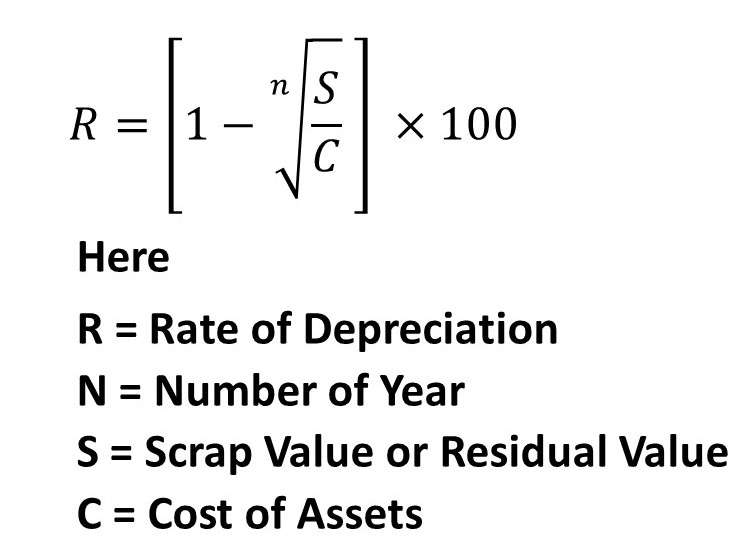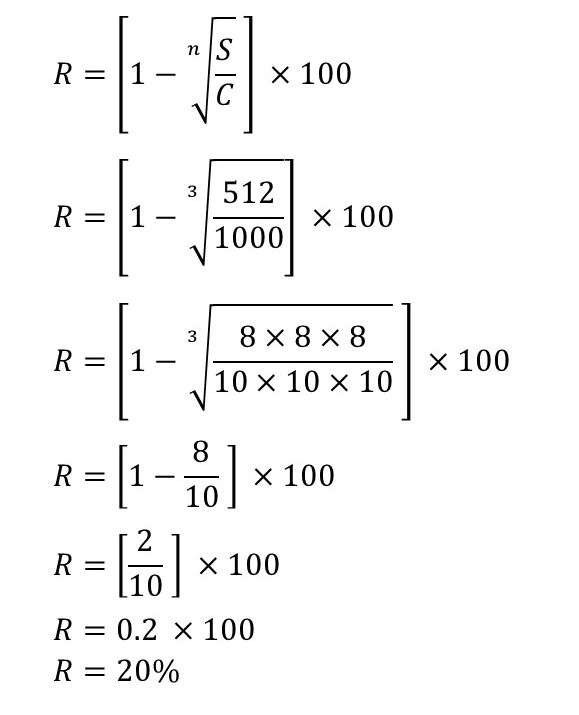# Diminishing Balance Method Or Reducing Balance Method Or Written Down Value Method

## Diminishing Balance Method:

Under this method, depreciation is charged as a fixed percentage on the book value of assets every year. In this method, the amount of depreciation decreases every year. This is a scientific method of charging depreciation because the cost of the assets is more in the initial years, then the amount depreciation is also more and as the value of the assets goes down then the amount of depreciation also decreases.

The amount of depreciation is calculated as a fixed percentage of the diminishing value of the asset shown in the books at the beginning of each year. Under this method, the value of an asset never comes to zero.

Suppose, the cost of the asset is 80,000 and the percentage to be written off each year is 10% by diminishing Balance method. In the first year, the amount of the depreciation will be 8,000 i.e., 10% of 80,000. This will reduce the book value to 72,000 i.e. 80,000 – 8,000.
Now, at the beginning of the next year, the book value is 72,000. The amount of the depreciation for the next year will be 7,200, i.e., 10% of 72,000. Thus, every year the amount of the depreciation will go on reducing. This method of charging depreciation is also known as Reducing Balance Method or Written Down ValueMethod.

### Characteristics of Diminishing Balance Method:

1. Depreciation is calculated on the opening balance of assets appearing in the books of the account.
2. The rate of depreciation remains the same every year.
3. The amount of depreciation goes on decreasing year by year.
4. It is a scientific method in the sense that with the decrease in the working capacity of an asset the amount of depreciation goes on decreasing.
5. Under this method, the value of an asset never comes to zero.
6. Diminishing balance method is also known as the reducing instalment method.
7. A higher percentage rate is needed to reduce the assets to their disposal value in a certain time period.

Methods of Charging Depreciation

### Merits of Diminishing Balance Method:

1. The calculation of depreciation is very easy.
2. The method is recognized by the Income Tax authorities.
3
. In the earlier days, when the amount of depreciation is higher, the repair will be less. On the other hand, in the latter days when the repairs are heavy, the depreciation will be less.
4. The diminishing balance method is suitable for costly and wearing assets as plants, machinery, motor lorry, etc.
5. Depreciation is charged according to the working capacity of the asset each year.

### Demerits of Diminishing Balance Method:

1. The value of the asset is not reduced to zero. There are some assets that remain of no use after a definite period.
2. It does not take the interest of capital invested for the asset into consideration.
3. If a low rate is ascertained for depreciation. It takes a very long time to write an asset off. Hence the rate has to be kept high.
4. There is no provision for the creation of funds to replace the depreciated asset under this method.
5. If an asset is sold or an additional asset is purchased during the accounting year, the calculation of profit or loss becomes a bit complicated.

### Calculation of Rate of Depreciation under Diminishing Balance Method:

When the cost of assets, estimated life of assets, and Scrap value or residual value are given, Depreciation can be calculated with the help of the following formula:Example:

X Company Ltd Purchased a Machinery ₹ 1,000. Estimated life of Machinery 3 Year. The Scrap value or residual value at the end of three years is expected to be ₹ 512. Depreciation charged by diminishing Balance method. Find out the Rate of Depreciation.

Solution: